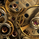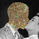913 obejrzeń
Estimating the LSMA Without Classics Parameters

I already mentioned various methods in order to estimate the LSMA in the idea i published. The parameter who still appeared on both the previous estimation and the classic LSMA was the sample correlation coefficient . This indicator will use an estimate of the correlation coefficient using the standard score thus providing a totally different approach in the estimation of the LSMA . My motivation for such indicator was to provide a different way to estimate a LSMA .

Standardization

The standard score is a statistical tool used to measure at how many standard deviations o a data point is bellow or above its mean. It can also be used to rescale variables, this conversion process is called standardizing or normalizing and it will be the basis of our estimation.

Calculation : (x - x̄)/o where is the moving average of x and o the standard deviation.

Estimating the Correlation Coefficient

We will use standardization to estimate the correlation coefficient r. 1 > r > -1 so in (y - x̄)/o we want to find y such that y is always above or below 1 standard deviation of , i had for first idea to pass the price through a band-stop filter but i found it was better to just use a moving average of period/2.

Estimating the LSMA

We finally rescale a line through the price like mentioned in my previous idea, for that we standardize a line and we multiply the result by our correlation estimation, next we multiply the previous calculation by the price standard deviation, then we sum this calculation to the price moving average.

Comparison of our estimate in white with a LSMA in red with both period 50 :

Working With Different Independents Variables

Here the independent variable is a line n (which represent the number of data point and thus create a straight line) but a classic LSMA can work with other independent variables, for exemple if a LSMA use the volume as independent variable we need to change our correlation estimate with (ȳ - x̄)/ô where ȳ is the moving average of period length/2 of y, y is equal to : change(close,length)*change( volume ,length) , is the moving average of y of period length, and ô is the standard deviation of y. This is quite rudimentary and if our goal is to provide a easier way to calculate correlation then the product-moment correlation coefficient would be more adapted (but less reactive than the sample correlation).

Conclusion

I showed a way to estimate the correlation coefficient , of course some tweaking could provide a better estimate but i find the result still quite close to the LSMA .

Patreon: https://www.patreon.com/alexgrover

You can also check out some of the indicators I made for luxalgo : https://www.tradingview.com/u/LuxAlgo/#published-scripts

## Komentarze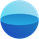Nice job! Your work keeps getting more and more impressive.
Odpowiedz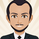alexgrover
@alexgrover, Hi professor, have you ever heard about Kriging and Gaussian process regression?
Odpowiedz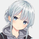everget
@everget, Thanks for your support, i have heard of Kriging but never used it. What kind of information do you need ?
Odpowiedzalexgrover
@alexgrover, I'm just wondering if the game is worth the candles if we talk about applying it to the financial time series.
Odpowiedz